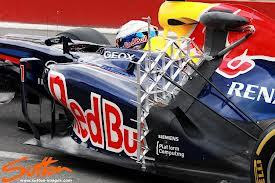### Boundary Layer considerationsFigure 1 – Lovely Heidelberg. I've been there. Birthplace of the boundary layer

When a body moves through the air, its interaction with the surrounding air mass generates a region where the air conditions are different from the freestream conditions. This region is named boundary layer. In basic air data applications there is a need to make accurate freestream conditions measurements, so we're really concerned about boundary layers. Boundary layers have been studied since the very beginning of aerodynamics. In 1904 a twenty-nine-year-old Ludwig Prandtl laid down the groundwork for modern aerodynamics with a short boundary layer presentation in Heidelberg .

Let's visualize the boundary layer over a flat plate, refer to Figure 2. We have depicted a laminar air flow, with vectors highlighting the velocity profile. The air flowing near the wall is slower than the air far from the wall, so the local velocity $$u(y)$$ is a function of the distance from wall $$y$$. The boundary layer is defined as the region where the local velocity values $$u(y)$$ are less or equal than the 99% value(or other reference value) of the freestream value $$u_{infty}$$. The speed at the wall is zero.Figure 2. Laminar flow boundary layer velocity profile

Air is viscous so to move one air layer with respect to other is necessary to apply a force.  Let's consider the flow (Couette flow) between two parallel flat plates of area $$A$$ at a distance $$y$$, one fixed to the ground and the other free to move. The force $$F$$ needed to maintain the speed $$u$$ of the moving plate is $$F=\mu Au/y$$. The newly introduced parameter $$\mu$$ is the air dynamic viscosity, with SI unit of measurement the $$Pa \cdot s$$. It is evident that energy is required to maintain a boundary layer of given characteristics between the two plates.

Video 1 – Turbulent and laminar flow

IIn general, boundary layers are affected by the relative speed of the fluid, the fluid properties and body geometry; see the following video that visualizes an example of laminar and turbulent flow.Figure 3 - Boundary layer velocity profiles in CFD pipe

The simplest way to visualize a boundary layer is to run a CFD simulation of a tube. You can find a tutorial here. From the figure 3 it is evident that the boundary layer is present and changes its shape along the pipe, the boundary changes along the length of the pipe, so the flow is said to be developing along the longitudinal axis. Let's suppose we want to calculate the flowrate across the pipe measuring the speed of the fluid at a single point inside the pipe. Flowrate is the integral over time of the velocity across a fixed section multiplied by the fluid density (Eq 12.2). To reconstruct the velocity values on the whole integration section based on a single measurement we need to know the analytical expression of the velocity profile. In many cases, that information can be gathered from precedent research work. It is evident that if we use the only measured velocity value as mean speed, neglecting the boundary layer, then we get a wrong flowrate measurement value.

Let's change the scenario and suppose that we want to know the true airspeed of a racing car, refer to figure 4. The issue here is that airspeed measured within the boundary layer is lower than the freestream airspeed. How far should our Pitot probe protrude from the car body to work properly? Visit this page for an analytical introductory approach.Figure 4 – Pitot rake on a race car

At a glance to deal with a boundary layer we need to know

• The boundary layer thickness
• The boundary layer velocity distribution and flow status: turbulent, laminar or transitional?
• Flow development patterns: where will the flow change from laminar to turbulent?

In our typical application we're dealing with a flying platform, where unfortunately the boundary layer characteristics change with the aircraft attitude and airspeed. For example the wing downwash affects all the posterior parts of the fuselage. The magnitude and pattern of the airflow are dependent on the angle of attack.

In the real world it is not trivial to find a position that is not affected by the aircraft attitude. To push the measurement performance further, CFD analysis, wind tunnel studies and in-flight calibration are needed.

Until now we focused our attention to the air velocity field, but the same definition of boundary layer can be used to define a temperature boundary layer. both boundary layers impact on basic air data measurements.

In the next post we will go into detail with a numerical example.